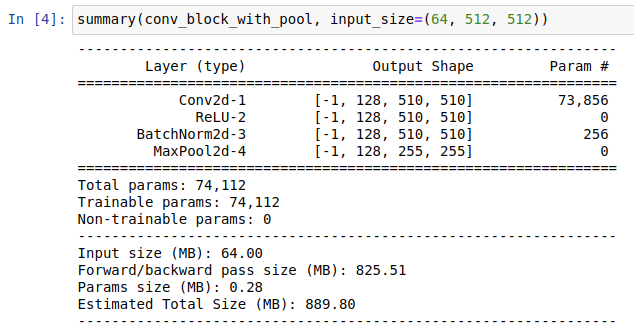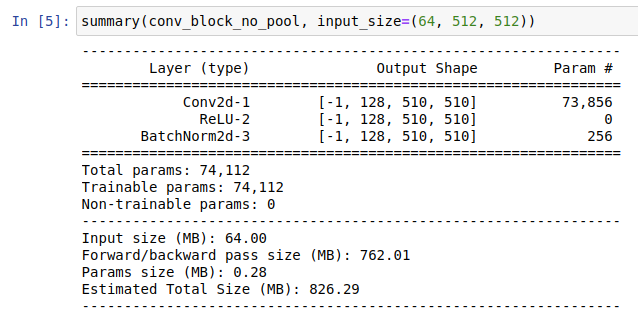# Do pooling layers use memory?

Hi,

I would like to ask if using pooling operations in the model definition results in extra memory usage. Imagine I define a simple set of operations as follows:

``````import torch
import torch.nn as nn
import torch.nn.functional as F

class ConvBlock(torch.nn.Module):
def __init__(self, in_channels, out_channels, pool=True):
super(ConvBlock, self).__init__()
self.pool = pool
blocks = []

blocks.append(nn.Conv2d(in_channels, out_channels, kernel_size=3))
blocks.append(nn.ReLU())
blocks.append(nn.BatchNorm2d(out_channels))

if self.pool:
blocks.append(nn.MaxPool2d(kernel_size = 2))

self.blocks = nn.Sequential(*blocks)

def forward(self, x):
out = self.blocks(x)
if not self.pool:
return F.max_pool2d(out, kernel_size=2)
return out

conv_block_with_pool = ConvBlock(64,128, pool=True)
conv_block_no_pool = ConvBlock(64,128, pool=False)
``````

It seems clear that both blocks are doing the same series of operations, only `conv_block_no_pool` applies pooling in the `forward` method rather than having it built on the definition.

I’m using torchsummary to measure memory usage. When using `conv_block_with_pool`, I obtain the following output:And when using `conv_block_no_pool` I get:Now, I am not sure if `torchsummary` is not measuring the whole thing in the second case, but I have read elsewhere that even if a layer has no parameters to be learned it still can take over some memory. Is this the case? And if so, should I always prefer/try to add this layers to my models in the forward method, rather than in the model definition itself?

Thanks,

Hi,

Yes pooling will take memory. But this is not the layer itself or its definition that uses the memory, but the fact that it introduces more intermediary results (that are then required to compute the gradients).
If you replaced the pooling with ` out = out + 1`, you will get similar thing.

The pooling might help though as the output will be smaller and the intermediary results of the next layers will be smaller as well.

Hi!

Thank you very much for your quick reply. Yes, I understand that, my point would rather be this question:

Does moving the pooling layer from the model definition (in the constructor) to the forward method bring any advantage in terms of memory consumption?

I guess this is probably a silly question (and the answer is no). I was just asking because if you look at the output from `torchsummary` that I was showing in my previous post, this would mean that the memory estimates that `torchsummary` gives are misleading in this case, and the memory consumed by both “models” would be exactly the same?

Thanks!

P.S.: While I have you here, may I also ask if there is any simple way of measuring/estimating the memory spent by a model in Pytorch while doing a forward/backward pass? Thanks